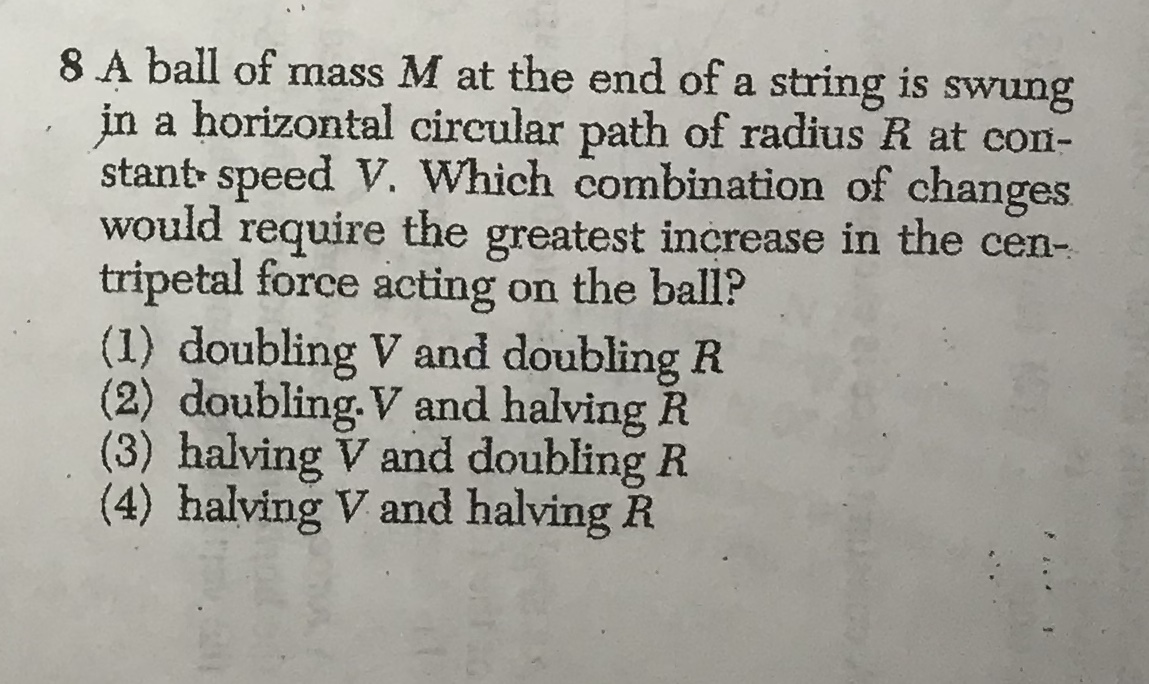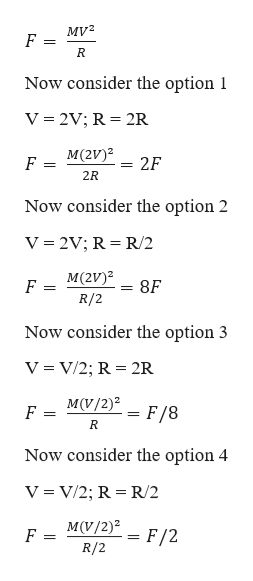# 8 A ball of mass M at the end of a string is swungin a horizontal circular path of radius R at con-stant speed V. Which combination of changeswouldrequiretripetal force acting on the ball?(1) doubling V and doubling R(2) doubling. V and halving R(3) halving V and doubling R(4) halving V and halving Rthegreatest increase in the cen-.

Question
398 viewshelp_outlineImage Transcriptionclose8 A ball of mass M at the end of a string is swung in a horizontal circular path of radius R at con- stant speed V. Which combination of changes would require tripetal force acting on the ball? (1) doubling V and doubling R (2) doubling. V and halving R (3) halving V and doubling R (4) halving V and halving R the greatest increase in the cen- . fullscreen
check_circle

Step 1

Given information:

Mass of the ball = M

Initial velocity of the ball = V

Radius of the path = R

Step 2

We know that centripetal ...help_outlineImage Transcriptioncloseму2 F R Now consider the option 1 V 2V; R 2R М(2V)2 F 2F 2R Now consider the option 2 V 2V; R R/2 М(2V)2 8F F R/2 Now consider the option 3 V = V/2; R 2R M(V/2) F = = F/8 R Now consider the option 4 V = V/2; R R/2 M(V/2) F/2 F R/2 fullscreen

### Want to see the full answer?

See Solution

#### Want to see this answer and more?

Solutions are written by subject experts who are available 24/7. Questions are typically answered within 1 hour.*

See Solution
*Response times may vary by subject and question.
Tagged in

### Angular Motion